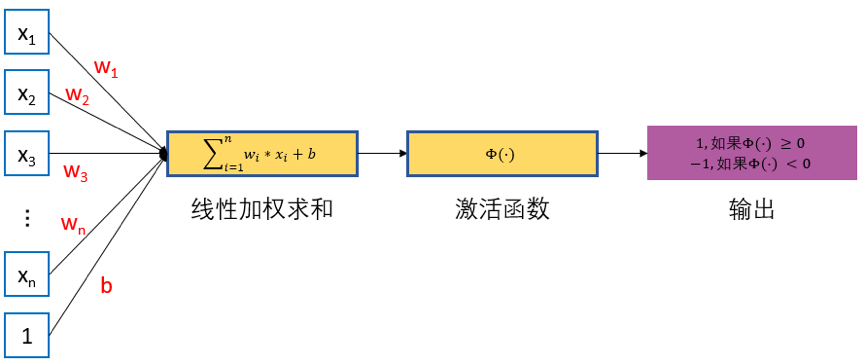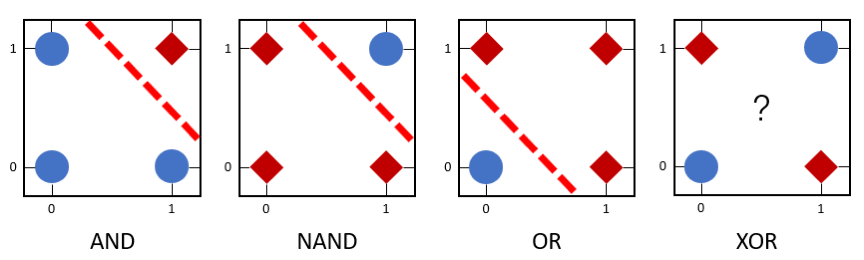# 单层感知机¶

## 1. 单层感知机模型¶

1957年 Frank Rosenblatt 提出了一种简单的人工神经网络，被称之为感知机。早期的感知机结构和 MCP 模型相似，由一个输入层和一个输出层构成，因此也被称为“单层感知机”。感知机的输入层负责接收实数值的输入向量，输出层则为1或-1两个值。单层感知机可作为一种二分类线性分类模型，结构如 图1 所示。$f(x) = sign(w*x+b)$

$\begin{split} sign(x) = \begin{cases} +1 \qquad & x \geq 0 \\ -1 \qquad & x \lt 0 \end{cases} \end{split}$

$$\varPhi(\cdot)$$会将 $$z$$ 与某一阈值（此例中，阈值为$$0$$）进行比较，如果大于等于该阈值则感知器输出为 $$1$$，否则输出为 $$-1$$。通过这样的操作，输入数据被分类为 $$1$$$$-1$$ 这两个不同类别。

## 2. 训练过程¶

1. 定义数据集，变量和参数，其中给定一个$$m*n$$大小的数据集，$$x^0, x^1,...,x^m$$ 为训练样本, $$x_0^m, x_1^m, ..., x_n^m$$为第 $$m$$ 条训练样本，$$d^m$$ 为期望结果，$$y^m$$ 为实际结果，$$\eta$$ 为学习率，$$0 \lt \eta \lt 1$$

2. 对权重系数$$w_j$$进行初始化，初始值为随机值或全零值。同时，设置 $$m = 0$$，读取第零条训练样本；

3. 将训练样本输入到单层感知机中，根据模型公式，得到实际输出 $$y$$ ;

4. 根据如下公式更新权重系数;

$w^{m+1} = w^m + \eta[d^m - y^m]x^m$
5. 当满足收敛条件时，算法结束；若不满足收敛条件则输入下一条样本继续训练，即 $$m = m +1$$。通常收敛条件可为：

• 误差小于某个预先设定的较小值 $$\epsilon$$ ;

• 迭代的权重系数间权值变化小于某个较小值；

• 迭代次数超过设定的最大迭代次数。

## 3. 单层感知机存在的问题¶